min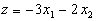s. t.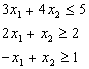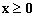3 4 1 0 0 0 0 5

2 1 0 -1 0 1 0 2

-1 1 0 0 -1 0 1 1

0 0 0 0 0 1 1 0

3 4 1 0 0 0 0 5

2 1 0 -1 0 1 0 2

-1 1 0 0 -1 0 1 1

-1 -2 0 1 1 0 0 -3

pivot on a32

7 0 1 0 4 0 -4 1

3 0 0 -1 1 1 -1 1

-1 1 0 0 -1 0 1 1

-3 0 0 1 -1 0 2 -1

pivot on a11

1.0000 0 0.1429 0 0.5714 0 -0.5714 0.1429

0 0 -0.4286 -1.0000 -0.7143 1.0000 0.7143 0.5714

0 1.0000 0.1429 0 -0.4286 0 0.4286 1.1429

0 0 0.4286 1.0000 0.7143 0 0.2857 -0.5714

The original is not feasible

b. The first and present simplex tableau are as follows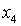2 c d 1 0 6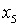-1 3 e 0 1 1

a 1 -2 0 0 0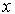g 2 -1 0.5 m f h i 1 0.5 1 p n 7 j k b 9

Find a to p.

SOLUTION

It is clear that at every iteration there will be at least two basic vectors with

1 at the basic variable and 0's else where therefore in the present tableau

the first and fifth vectors are the basic vectors. Now since 1 is in 5th vector

then the rest is zero i.e.

m=b=0.

Also the first vector is basic this implies

g=1,h=n=0.

Using the simplex method in matrix form i.e.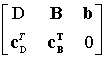=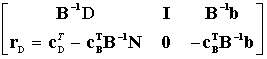Now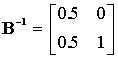then using the above six equality you will find that

a=-3,k=1.5,c=4,j=-5i=5,e=0,d=-2,f=3,p=4.